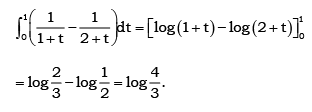# Test: Definite And Indefinite Integral (Competition Level)

## 30 Questions MCQ Test Mathematics (Maths) Class 12 | Test: Definite And Indefinite Integral (Competition Level)

Description
This mock test of Test: Definite And Indefinite Integral (Competition Level) for JEE helps you for every JEE entrance exam. This contains 30 Multiple Choice Questions for JEE Test: Definite And Indefinite Integral (Competition Level) (mcq) to study with solutions a complete question bank. The solved questions answers in this Test: Definite And Indefinite Integral (Competition Level) quiz give you a good mix of easy questions and tough questions. JEE students definitely take this Test: Definite And Indefinite Integral (Competition Level) exercise for a better result in the exam. You can find other Test: Definite And Indefinite Integral (Competition Level) extra questions, long questions & short questions for JEE on EduRev as well by searching above.
QUESTION: 1

### Let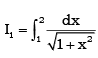and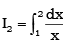then

Solution:

We have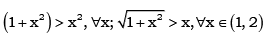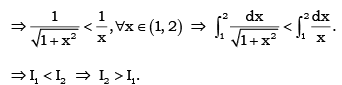QUESTION: 2

###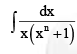is equal to

Solution: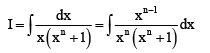Putting xn = t so that n xn–1 dx = dt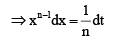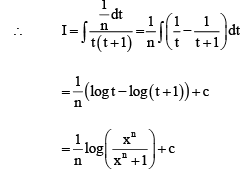QUESTION: 3

###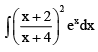is equal to

Solution: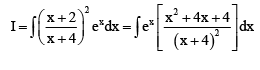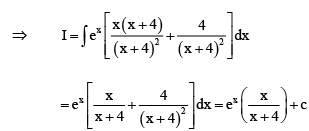QUESTION: 4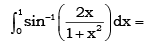Solution: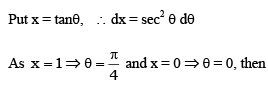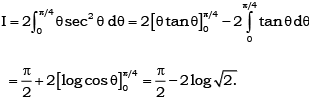QUESTION: 5

If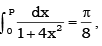then P =

Solution: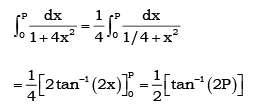Comparing it with the given value, we get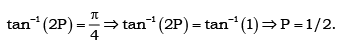QUESTION: 6

The value of integral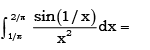Solution:

put t = 1/x ⇒ dt = -1/x2 as t = π/2 and π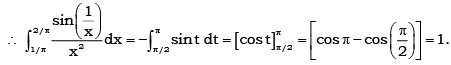QUESTION: 7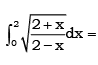Solution:

Put x = 2 cos θ ⇒ dx = - 2 sin θ dθ, then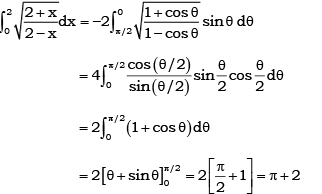QUESTION: 8

If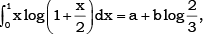then

Solution:

Integrate it by parts taking  log (1+ x/2 )as first function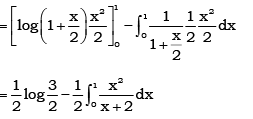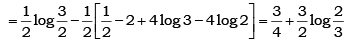QUESTION: 9

The value of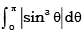is

Solution: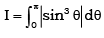Since sinq is positive in interval (0, π)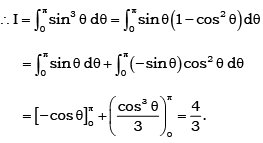QUESTION: 10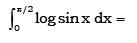Solution: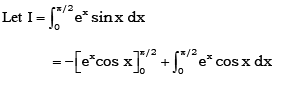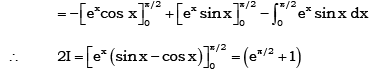QUESTION: 11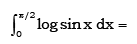Solution: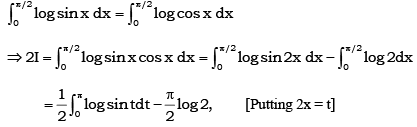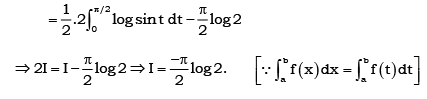QUESTION: 12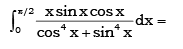Solution: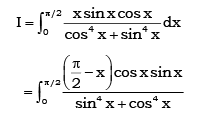By adding (i) and (ii), we get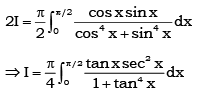Now, Put tan2x = t, we get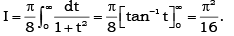QUESTION: 13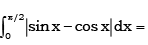Solution: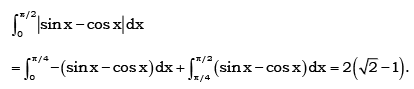QUESTION: 14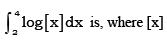denotes the greater integer less than or equal to x

Solution: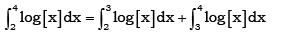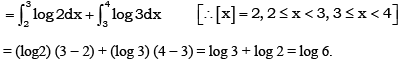QUESTION: 15

If [x] denotes the greater integer less than or equal to x, then the value of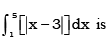Solution: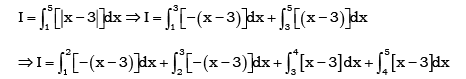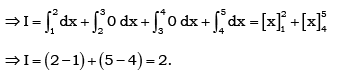QUESTION: 16

If f(x) = tan x - tan3 x + tan5 x - …… to ∞ with 0 < x < π/4, then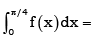Solution: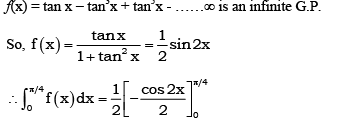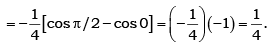QUESTION: 17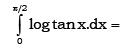Solution:

I = ∫0 π2 log(tan x).dx
I = ∫0 π2 log(cot x).dx
Adding both the equations, we get
2I = ∫0 π2 log(tanx) + log(cot x) dx
2I = ∫0 π2 log(1).dx
= 0

QUESTION: 18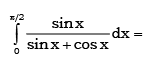Solution: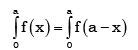QUESTION: 19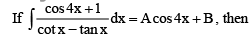Solution: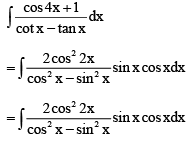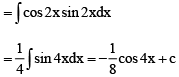QUESTION: 20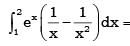Solution:

f’(x) = -1/x2
Thus, ∫(1 to 2)ex(1/x - 1/x2)dx
= [ex/x](1 to 2) + c
= e2/2 - e

QUESTION: 21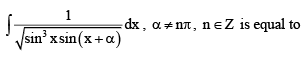Solution: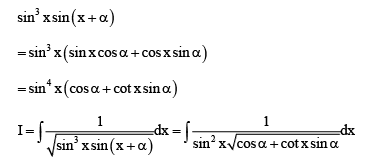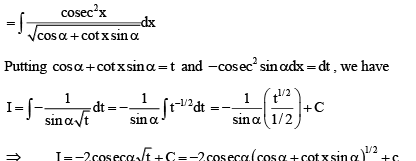QUESTION: 22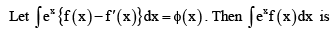Solution:

Here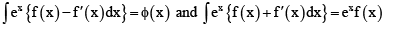on adding we get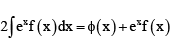QUESTION: 23

If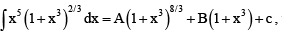then

Solution: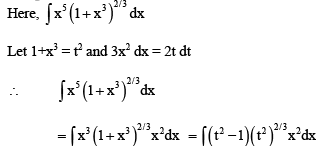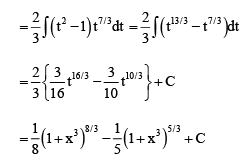QUESTION: 24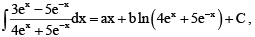then

Solution: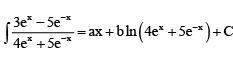Differentiating both sides, we get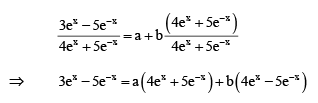Comparing the coefficient of like terms on both sides, we get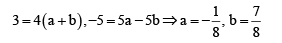QUESTION: 25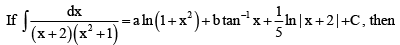Solution: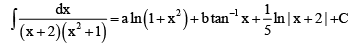Differentiating both sides, we get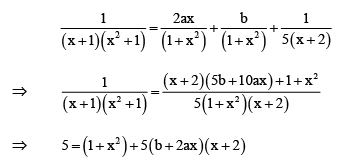Comparing the like powers of x in both sides, we get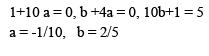QUESTION: 26

If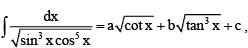then

Solution: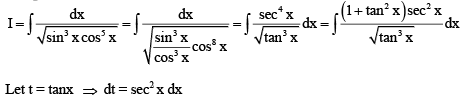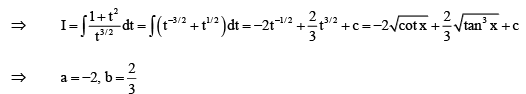QUESTION: 27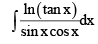is equal to

Solution:

t = ln(tan x)
dt = (sec2 x)/(tan x) dx
=> (1/cos^2x) * (cosx /sinx) dx = dt
dt = dx/(cosx sinx)
I = ∫t dt
= [t2]/2 + c
= 1/2[ln(tanx)]2 + c

QUESTION: 28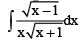is equal to

Solution: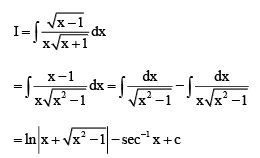QUESTION: 29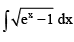is equal to

Solution: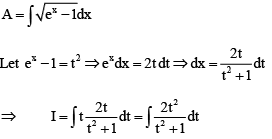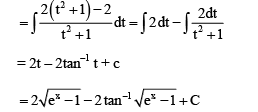QUESTION: 30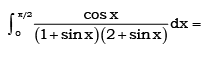Solution:

ut sin x = t Þ cos x dx = dt, so that reduced integral is